## Class 11 Important Questions for Chemistry – Organic Chemistry

NCERT Exemplar Class 11 Chemistry is very important resource for students preparing for XI Board Examination. Here we have provided NCERT Exemplar Problems Solutions along with NCERT Exemplar Problems Class 11.

Question from very important topics are covered by NCERT Exemplar Class 11. You also get idea about the type of questions and method to answer in your Class 11th examination.

for Class 11 Chemistry Notes

Here you can get Class 11 Important Questions Chemistry based on NCERT Text book for Class XI. Chemistry Class 11 Important Questions are very helpful to score high marks in board exams. Here we have covered Important Questions on Organic Chemistry for Class 11 Chemistry subject.

Chemistry Important Questions Class 11 are given below.

### Multiple Choice Questions (Type-I)

1. Which of the following is the correct IUPAC name?
• (i) 3-Ethyl-4, 4-dimethylheptane
• (ii) 4,4-Dimethyl-3-ethylheptane
• (iii) 5-Ethyl-4, 4-dimethylheptane
• (iv) 4,4-Bis(methyl)-3-ethylheptane
2.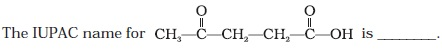• (i) 1-hydroxypentane-1,4-dione
• (ii) 1,4-dioxopentanol
• (iii) 1-carboxybutan-3-one
• (iv) 4-oxopentanoic acid
3. The IUPAC name for• (i) 1-Chloro-2-nitro-4-methylbenzene
• (ii) 1-Chloro-4-methyl-2-nitrobenzene
• (iii) 2-Chloro-1-nitro-5-methylbenzene
• (iv) m-Nitro-p-chlorotoluene
4. Electronegativity of carbon atoms depends upon their state of hybridisation. In which of the following compounds, the carbon marked with asterisk is most electronegative?
• (i) CH3 – CH2 – *CH3 –CH3
• (ii) CH3 – *CH = CH – CH3
• (iii) CH3 – CH2 – C ≡ *CH
• (iv) CH3 – CH2 – CH = *CH2
5. In which of the following, functional group isomerism is not possible?
• (i) Alcohols
• (ii) Aldehydes
• (iii) Alkyl halides
• (iv) Cyanides
6. The fragrance of flowers is due to the presence of some steam volatile organic compounds called essential oils. These are generally insoluble in water at room temperature but are miscible with water vapour in vapour phase. A suitable method for the extraction of these oils from the flowers is:
• (i) Distillation
• (ii) Crystallisation
• (iii) Distillation under reduced pressure
• (iv) Steam distillation
7. During hearing of a court case, the judge suspected that some changes in the documents had been carried out. He asked the forensic department to check the ink used at two different places. According to you which technique can give the best results?
• (i) Column chromatography
• (ii) Solvent extraction
• (iii) Distillation
• (iv) Thin layer chromatography
8. The principle involved in paper chromatography is
• (i) Adsorption
• (ii) Partition
• (iii) Solubility
• (iv) Volatility
9. What is the correct order of decreasing stability of the following cations.• (i) II > I > III
• (ii) II > III > I
• (iii) III > I > II
• (iv) I > II > III
10.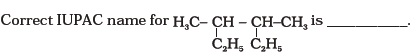• (i) 2- ethyl-3-methylpentane
• (ii) 3,4- dimethylhexane
• (iii) 2-sec-butylbutane
• (iv) 2, 3-dimethylbutane
11. In which of the following compounds the carbon marked with asterisk is expected to have greatest positive charge?
• (i) *CH3 —CH2 —Cl
• (ii) *CH3 —CH2 —Mg+Cl
• (iii) *CH3 —CH2 —Br
• (iv) *CH3 —CH2 —CH3
12. Ionic species are stabilised by the dispersal of charge. Which of the following carboxylate ion is the most stable?13. Electrophilic addition reactions proceed in two steps. The first step involves the addition of an electrophile. Name the type of intermediate formed in the first step of the following addition reaction.
H3C—HC = CH2 + H + ⎯→?
• (i) 2° Carbanion
• (ii) 1° Carbocation
• (iii) 2° Carbocation
• (iv) 1° Carbanion
14.  Covalent bond can undergo fission in two different ways. The correct representation involving a heterolytic fission of CH3 —Br is15.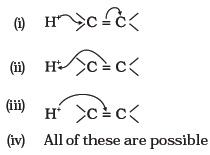### Multiple Choice Questions (Type-II)

In the following questions two or more options may be correct.

1. Which of the following compounds contain all the carbon atoms in the same hybridisation state?
• (i) H—C ≡ C—C ≡ C—H
• (ii) CH3 —C ≡ C—CH3
• (iii) CH2 = C = CH2
• (iv) CH2 = CH—CH = CH2
2. In which of the following representations given below spatial arrangement of group/ atom different from that given in structure ‘A’?3. Electrophiles are electron seeking species. Which of the following groups contain only electrophiles?Note : Consider the following four compounds for answering questions 19 and 20.4. Which of the following pairs are position isomers?
• (i) I and II
• (ii) II and III
• (iii) II and IV
• (iv) III and IV
5. Which of the following pairs are not functional group isomers?
• (i) II and III
• (ii) II and IV
• (iii) I and IV
• (iv) I and II
6. Nucleophile is a species that should have
• (i) a pair of electrons to donate
• (ii) positive charge
• (iii) negative charge
• (iv) electron deficient species
7. Hyperconjugation involves delocalisation of ___________.
• (i) electrons of carbon-hydrogen σ bond of an alkyl group directly attached to an atom of unsaturated system.
• (ii) electrons of carbon-hydrogen σ bond of alkyl group directly attached to the positively charged carbon atom.
• (iii) π-electrons of carbon-carbon bond
• (iv) lone pair of electrons

### Short Answer Type Questions

Note : Consider structures I to VII and answer the questions 23–26.1. Which of the above compounds form pairs of metamers?
2. Identify the pairs of compounds which are functional group isomers.
3. Identify the pairs of compounds that represents position isomerism.
4. Identify the pairs of compounds that represents chain isomerism.
5. For testing halogens in an organic compound with AgNO3 solution, sodium extract (Lassaigne’s test) is acidified with dilute HNO3. What will happen if a student acidifies the extract with dilute H2SO4 in place of dilute HNO3?
6. What is the hybridisation of each carbon in H2C = C = CH2.
7. Explain, how is the electronegativity of carbon atoms related to their state of hybridisation in an organic compound?
8. Show the polarisation of carbon-magnesium bond in the following structure.
CH3—CH2—CH2—CH2—Mg—X
9. Compounds with same molecular formula but differing in their structures are said to be structural isomers. What type of structural isomerism is shown by10. Which of the following selected chains is correct to name the given compound according to IUPAC system.11. In DNA and RNA, nitrogen atom is present in the ring system. Can Kjeldahl method be used for the estimation of nitrogen present in these? Give reasons.
12. If a liquid compound decomposes at its boiling point, which method (s) can you choose for its purification. It is known that the compound is stable at low pressure, steam volatile and insoluble in water.
Note : Answer the questions 35 to 38 on the basis of information given below:
“Stability of carbocations depends upon the electron releasing inductive effect of groups adjacent to positively charged carbon atom involvement of neighbouring groups in hyperconjugation and resonance.”
13.14. Which of the following ions is more stable? Use resonance to explain your answer.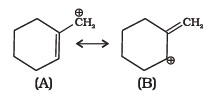15. The structure of triphenylmethyl cation is given below. This is very stable and some of its salts can be stored for months. Explain the cause of high stability of this cation.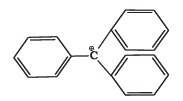16. Write structures of various carbocations that can be obtained from 2-methylbutane. Arrange these carbocations in order of increasing stability.
17. Three students, Manish, Ramesh and Rajni were determining the extra elements present in an organic compound given by their teacher. They prepared the Lassaigne’s extract (L.E.) independently by the fusion of the compound with sodium metal. Then they added solid FeSO4 and dilute sulphuric acid to a part of Lassaigne’s extract. Manish and Rajni obtained prussian blue colour but Ramesh got red colour. Ramesh repeated the test with the same Lassaigne’s extract, but again got red colour only. They were surprised and went to their teacher and told him about their observation. Teacher asked them to think over the reason for this. Can you help them by giving the reason for this observation. Also, write the chemical equations to explain the formation of compounds of different colours.
18. Name the compounds whose line formulae are given below :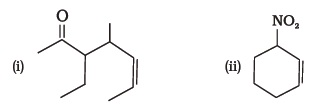19. Write structural formulae for compounds named as-
• (a) 1-Bromoheptane
• (b) 5-Bromoheptanoic acid
20. Draw the resonance structures of the following compounds;21. Identify the most stable species in the following set of ions giving reasons :22. Give three points of differences between inductive effect and resonance effect.
23. Which of the following compounds will not exist as resonance hybrid. Give reason for your answer :
• (i) CH3 OH
• (ii) R—CONH2
• (iii) CH3CH = CHCH2NH2
24. Why does SO3 act as an electrophile?
25. Resonance structures of propenal are given below. Which of these resonating structures is more stable? Give reason for your answer.26. By mistake, an alcohol (boiling point 97°C) was mixed with a hydrocarbon (boiling point 68°C). Suggest a suitable method to separate the two compounds. Explain the reason for your choice.
27. Which of the two structures (A) and (B) given below is more stabilised by resonance? Explain.### Matching Type Questions

In the following questions more than one correlation is possible between options of Column I and Column II. Make as many correlations as you can.

1. Match the type of mixture of compounds in Column I with the technique of separation/purification given in Column II.2. Match the terms mentioned in Column I with the terms in Column II.3. Match Column I with Column II.4. Match the intermediates given in Column I with their probable structure in Column II.5. Match the ions given in Column I with their nature given in Column II.### Assertion and Reason Type Questions

In the following questions a statement of Assertion (A) followed by a statement of Reason (R) is given. Choose the correct option out of the choices given below each question.

1. Assertion (A) : Simple distillation can help in separating a mixture of propan-1-ol (boiling point 97°C) and propanone (boiling point 56°C).
Reason (R) : Liquids with a difference of more than 20°C in their boiling points can be separated by simple distillation.
• (i) Both A and R are correct and R is the correct explanation of A.
• (ii) Both A and R are correct but R is not the correct explanation of A.
• (iii) Both A and R are not correct.
• (iv) A is not correct but R is correct.
2. Assertion (A) : Energy of resonance hybrid is equal to the average of energies of all canonical forms.
Reason (R) : Resonance hybrid cannot be presented by a single structure.
• (i) Both A and R are correct and R is the correct explanation of A.
• (ii) Both A and R are correct but R is not the correct explanation of A.
• (iii) Both A and R are not correct.
• (iv) A is not correct but R is correct.
3. Assertion (A) : Pent- 1- ene and pent- 2- ene are position isomers.
Reason (R) : Position isomers differ in the position of functional group or a substituent.
• (i) Both A and R are correct and R is the correct explanation of A.
• (ii) Both A and R are correct but R is not the correct explanation of A.
• (iii) Both A and R are not correct.
• (iv) A is not correct but R is correct.
4. Assertion (A) : All the carbon atoms in H 2 C = C = CH 2 are sp 2 hybridised
Reason (R) : In this molecule all the carbon atoms are attached to each other by double bonds.
• (i) Both A and R are correct and R is the correct explanation of A.
• (ii) Both A and R are correct but R is not the correct explanation of A.
• (iii) Both A and R are not correct.
• (iv) A is not correct but R is correct.
5. Assertion (A) : Sulphur present in an organic compound can be estimated quantitatively by Carius method.
Reason (R) : Sulphur is separated easily from other atoms in the molecule and gets precipitated as light yellow solid.
• (i) Both A and R are correct and R is the correct explanation of A.
• (ii) Both A and R are correct but R is not the correct explanation of A.
• (iii) Both A and R are not correct.
• (iv) A is not correct but R is correct.
6. Assertion (A): Components of a mixture of red and blue inks can be separated by distributing the components between stationary and mobile phases in paper chromatography.
Reason (R) : The coloured components of inks migrate at different rates because paper selectively retains different components according to the difference in their partition between the two phases.
• (i) Both A and R are correct and R is the correct explanation of A.
• (ii) Both A and R are correct but R is not the correct explanation of A.
• (iii) Both A and R are not correct.
• (iv) A is not correct but R is correct.

### Long Answer Type Questions

1. What is meant by hybridisation? Compound CH2 = C = CH2 contains sp or sp2 hybridised carbon atoms. Will it be a planar molecule?
2. Benzoic acid is a organic compound. Its crude sample can be purified by crystallisation from hot water. What characteristic differences in the properties of benzoic acid and the impurity make this process of purification suitable?
3. Two liquids (A) and (B) can be separated by the method of fractional distillation. The boiling point of liquid (A) is less than boiling point of liquid (B). Which of the liquids do you expect to come out first in the distillate? Explain.
4. You have a mixture of three liquids A, B and C. There is a large difference in the boiling points of A and rest of the two liquids i.e., B and C. Boiling point of liquids B and C are quite close. Liquid A boils at a higher temperature than B and C and boiling point of B is lower than C. How will you separate the
components of the mixture. Draw a diagram showing set up of the apparatus for the process.
5. Draw a diagram of bubble plate type fractionating column. When do we require such type of a column for separating two liquids. Explain the principle involved in the separation of components of a mixture of liquids by using fractionating column. What industrial applications does this process have?
6. A liquid with high boiling point decomposes on simple distillation but it can be steam distilled for its purification. Explain how is it possible?
 « Previous Next »

## Answers to Multiple Choice Questions

 MCQ (Type-I) Q.No. Answer Q.No. Answer Q.No. Answer Q.No. Answer Q.No. Answer Q.No. Answer 1 (ii) 2 (iv) 3 (ii) 4 (iii) 5 (iii) 6 (iv) 7 (iv) 8 (ii) 9 (i) 10 (ii) 11 (i) 12 (iv) 13 (iii) 14 (ii) 15 (ii)
 MCQ (Type-II) Q.No. Answer Q.No. Answer Q.No. Answer 1 (i), (iv) 2 (i), (iii), (iv) 3 (ii), (iii) 4 (ii) 5 (i), (iii) 6 (i), (iii) 7 (i), (ii)